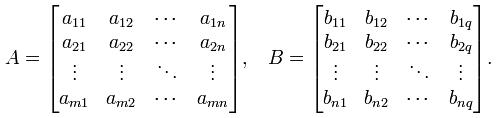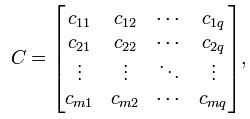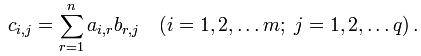favorite We need a little bit of your help to keep things running, click on this banner to learn more
Problems

# Matrix multiplication

Given two square matrices A and B of dimension m × n and n × q respectively:Then the matrix C of dimension m × q is called their product:where:The operation of multiplication of two matrices is feasible only if the number of columns in the first factor equals to the number of rows in the second. In this case we say that the shape of the matrices is consistent.

Given two matrices A and B. Find their product.

#### Input

The first line contains two positive integers `na` and `ma` - the dimensions of matrix A. Each of the next `na` rows contains `ma` numbers - the elements `aij` of matrix A. In (`na` + 2)-nd row two positive integers `nb` and `mb` are given - the dimensions of matrix B. In the following `nb` lines `mb` numbers are given - the elements `bij` of matrix B. Dimensions of the matrices do not exceed 100 × 100, all integers do not exceed 100 by absolute value.

#### Output

Print in the first line the dimensions of the resulting matrix C: `nс` and `mc`. In the next `nс` rows print space separated `mc` numbers - the corresponding elements `cij` of matrix C. If you can not multiply matrixs in the first and only line of output -1.

Time limit 1 seconds
Memory limit 128 MiB
Input example #1
```2 3
1 3 4
5 -2 3
3 3
1 3 2
2 1 3
0 -1 1
```
Output example #1
```2 3
7 2 15
1 10 7
```